Courses

# Systems of Linear Equations - Matrices and Determinants, Business Mathematics & Statistics B Com Notes | EduRev

## B Com : Systems of Linear Equations - Matrices and Determinants, Business Mathematics & Statistics B Com Notes | EduRev

The document Systems of Linear Equations - Matrices and Determinants, Business Mathematics & Statistics B Com Notes | EduRev is a part of the B Com Course Business Mathematics and Statistics.
All you need of B Com at this link: B Com

SYSTEMS OF LINEAR EQUATIONS

Submatrices and minors of amatrix.

Matrices obtained from a given matrix A by omitting some of its rows and columns are called sub matrices of A.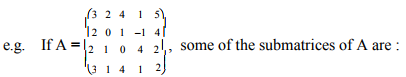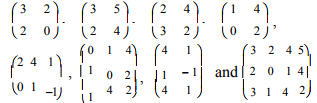The determinants of the square submatrices are calledminors of the matrix.
Some of the minors of A are :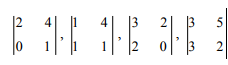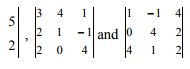Rank of a matrix.
A positive integer ‘r’ is said to be the rank of a non zero matrix A, denoted by ρ(A), if
(i) there is at least one minor of A of order‘r’ which is not zero and
(ii) every minor of A of order greater than ‘r’ is zero.

Note
(i) The rank of a matrix A is the order of the largest non zero minor of A.
(ii) If A is a matrix of order m x n then ρ(A) ≤ 3 minimum (m, n)
(iii) The rank of a zero matrix is taken to be 0.
(iv) For non zero matrices, the least value of the rank is 1.
(v) The rank of a non singular matrix of order n x n is n.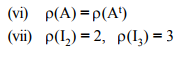Example 7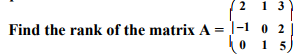Solution : Order of A is 3 x 3. ∴ ρ(A)≤3 Consider the only third order minor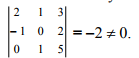There is a minor of order 3 which is not zero. ∴ ρ(A) =3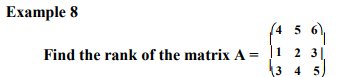Solution :

Order of A is 3 x 3. ρ(A) ≤ 3 Consider the only third order minor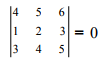The only minor of order 3 is zero. ∴ ρ(A)≤2 Consider the second order minors.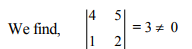There is a minor of order 2 which is non zero.   ∴ ρ(A)≤2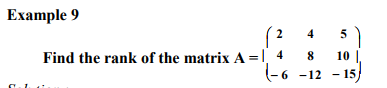Solution : Order of A is 3 x 3 ∴ ρ(A)≤ 3 Consider the only third order minor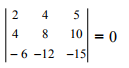(R1 ∞R2 )

The only minor of order 3 is zero.    ∴ ρ(A)≤ 2
Consider the second order minors. Obviously they are all zero.
ρ(A)≤ 1 Since A is a non zero matrix, ρ(A) =1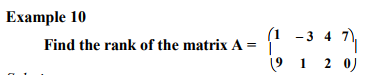Solution : Order of A is 2 x 4.        ∴ ρ(A)≤ 2
Consider the second order minors.
We find,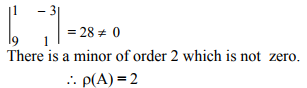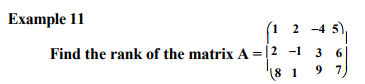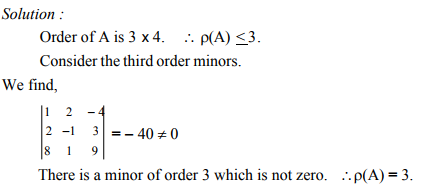Elementary operations and equivalent matrices. The process of finding the values of a number of minors in our endeavour to find the rank of a matrix becomeslaborious unless by a stroke of luck we get a non zero minor at an early stage. To get over this difficulty, we introduce many zeros in the matrix by what are called elementary operations so that the evaluation of the minors is rendered easier. It can be proved that the elementary operations do not alter the rank of a matrix.
The following are the elementary operations :
(i) The interchange of two rows.
(ii) The multiplication of a row by a non zero number.
(iii) The addition of a multiple of one row to another row. If a matrix B is obtained from a matrix A by a finite number of elementary operations then we say that the matrices A and B are equivalent matrices and we write A ~ B.
Also, while introducing many zeros in the given matrix, it would be desirable (but not necessary) to reduce it to a triangular form.

A matrix A = (aij ) is said to be in a triangular form if aij = 0 whenever i > j.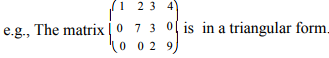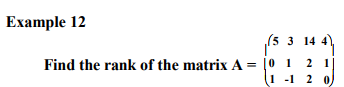Solution : Order of A is 3 x 4.       ∴ ρ(A)≤ 2

Let us reduce the matrix A to a triangular form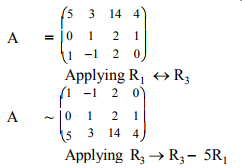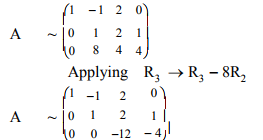This is now in a triangular form.
We find,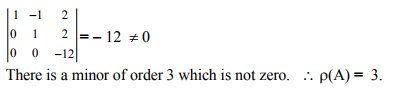Example 13  Find the rank of the matrix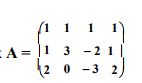Solution : Order of A is 3 x 4.         ∴ ρ(A)≤ 3

Let us reduce the matrix A to a triangular form.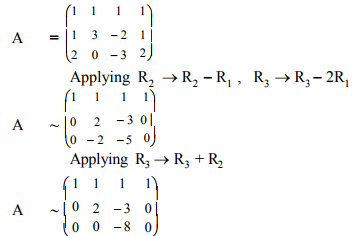This is now in a triangular form. We find,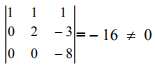There is a minor of order 3 which is not zero.    ∴ ρ(A)≤ 3

Example 14 Find the rank of the matrix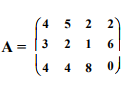Solution : Order of A is 3 x 4.           ∴ ρ(A)≤ 3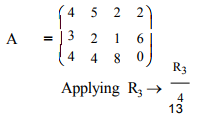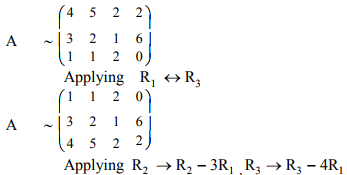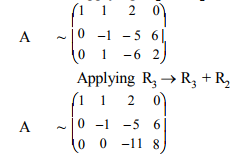This is in a triangular form.
We find,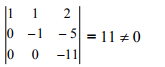There is a minor of order 3 which is not zero.          ∴ ρ(A)≤ 3

Systems of linear equations.
A system of (simultaneous) equations in which the variables (ie. the unknowns) occur only in the first degree is said to be linear.

A system of linear equations can be represented in the form AX = B. For example, the equations x-3y+z = -1, 2x+y-4z =-1, 6x-7y+8z = 7 can be written in the matrix form as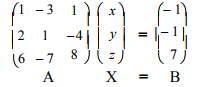A is called the coefficient matrix. If the matrix A is augmented with the column matrix B, at the end, we get the augmented matrix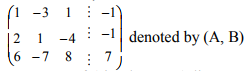A system of (simultaneous) linear equations is said to be homogeneous if the constant term in each of the equations is zero. A system of linear homogeneous equations can be represented in the form AX = O. For example, the equations 3x+4y-2z = 0, 5x+2y = 0, 3x-y+z = 0 can be written in the matrix form as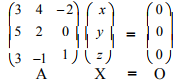Consistency of equations
A system of equations is said to be consistent if it has at least one set of solution. Otherwise it is said to be inconsistent.
Consistent equations may have
(i) unique solution (that is, only one set of solution) or
(ii) infinite sets of solution.

By way of illustration, consider first the case of linear equations in two variables.

The equations 4x- y = 8, 2x + y = 10 represent two straight lines intersecting at (3, 4). They are consistent and have the unique solution x = 3, y = 4. (Fig. 1.1)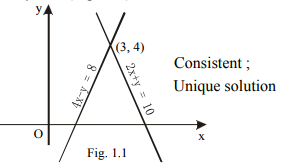The equations 5x - y = 15, 10x - 2y = 30 represent two coincident lines. We find that any point on the line is a solution. The equations are consistent and have infinite sets of solution such as x = 1, y = -10 ; x = 3, y = 0 ; x = 4, y = 5 and so on (Fig. 1.2) Such equations are called dependent equations.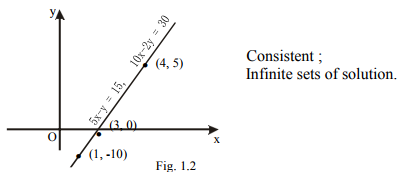The equations 4x - y = 4 , 8x - 2y = 5 represent two parallel straight lines. The equations are inconsistent and have no solution. (Fig. 1.3)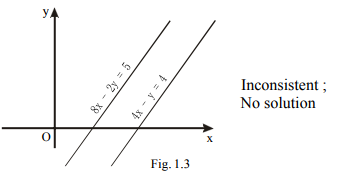Now consider the case of linear equations in three variables. The equations 2x + 4y + z = 5, x + y + z = 6, 2x + 3y + z = 6 are consistent and have only one set of unique solution viz. x = 2, y = -1, z = 5. On the other hand, the equations x + y + z = 1, x + 2y + 4z = 1, x + 4y + 10z = 1 are consistent and have infinite sets ofsolution such as x = 1, y = 0, z = 0 ; x = 3, y = -3, z = 1 ; and so on. All these solutions are included in x = 1+2k , y = -3k, z = k where k is a parameter.

The equations x + y + z = -3, 3x + y - 2z = -2, 2x +4y + 7z = 7 do not have even a single set of solution. They are inconsistent.

All homogeneous equations do have the trivial solution x = 0, y = 0, z = 0. Hence the homogeneous equations are all consistent and the question of their being consistent or otherwise does not arise at all.

The homogeneous equations may or may not have solutions other than the trivial solution. For example, the equations x + 2y + 2z = 0, x -3y -3z = 0, 2 x +y -z = 0 have only the trivial solution viz., x = 0, y = 0, z = 0. On the other hand the equations x +y -z = 0, x -2y +z = 0, 3x +6y -5z = 0 have infinite sets of solution such as x = 1, y = 2, z = 3 ; x = 3, y = 6, z = 9 and so on. All these non trivial solutions areincluded in x = t, y = 2t, z = 3t where t is a parameter.

Testing the consistency of equations by rank method.
Consider the equations AX = B in 'n' unknowns
1) If ρ(A, B) = ρ(A), then the equations are consistent.
2) If ρ(A, B)   ρ(A), then the equations are inconsistent.
3) If ρ(A, B) = ρ(A) = n , then the equations are consistentand have unique solution.
4) If ρ(A, B) = ρ(A) < n , then the equations are consistentand have infinite sets of solution.

Consider the equations AX = 0 in 'n' unkowns
1) If ρ(A) = n then equations have the trivial solution only.
2) If ρ(A) < n then equations have the non trivial solutions also.

Example 15
Show that the equations 2 x - y +z = 7, 3 x +y- 5z = 13, x +y +z = 5 are consistent and have unique solution.

Solution : The equations take the matrix form as

Obviously,

ρ(A, B) = 3, ρ(A) = 3

The number of unknowns is 3.
Hence ρ(A, B) = ρ(A) = the number of unknowns.

∴ The equations are consistent and have unique solution.

Example 16 Show that the equations x + 2y = 3, y - z = 2, x + y + z = 1 are consistent and have infinite sets of solution
Solution : The equations take the matrix form as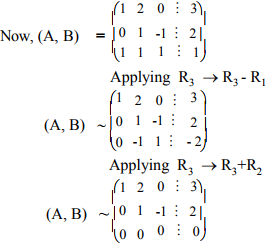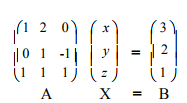Obviously, ρ(A, B) = 2, ρ(A) = 2.
The number of unknowns is 3.
Hence ρ(A, B) = ρ(A) < the number of unknowns.
∴ The equations are consistent and have infinite sets of solution.

Example 17 Show that the equations x -3y +4z = 3, 2x -5y +7z = 6, 3x -8y +11z = 1 are inconsistent.

Solution : The equations take the matrix form as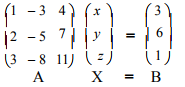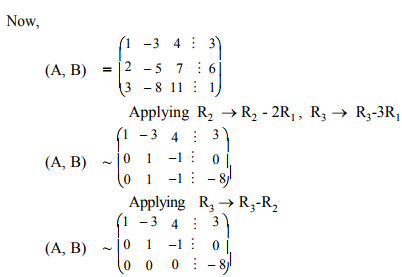Obviously,
ρ(A, B) = 3,ρA) = 2
Hence ρ(A, B) ≠ ρ(A)

∴ The equations are inconsistent.

Exampe 18 Show that the equations x +y +z = 0, 2x +y- z = 0, x - 2y +z = 0 have only the trivial solution.

Solution : The matrix form of the equations is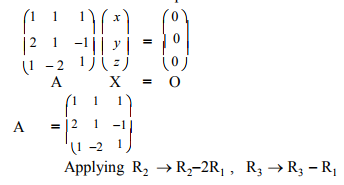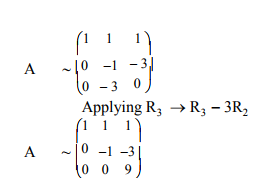Obviously,ρ(A) = 3
The number of unknowns is 3.
Hence  ρ(A) = the number of unknowns.
∴ The equations have only the trivial solution.

Example 19 Show that the equations 3x +y + 9z = 0, 3x +2y + 12z =0, 2x +y + 7z = 0 have non trivial solutions also.

Solution :

The matrix form of the equations is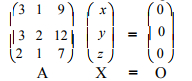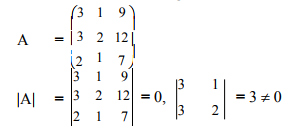∴  ρ(A)  = 2 3 = 0, 3 1 = 3  0 2
The number of unknowns is 3.
Hence ρ(A) < the number of unknowns.
∴ The equations have non trivial solutions also

Example 20 Find k if the equations 2x + 3y -z = 5, 3x -y + 4z = 2, x + 7y -6z = k are consistent.

Solution :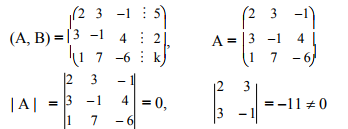Obviously ρ(A) = 2.
For the equations to be consistent, ρ(A, B) should also be 2.
Hence every minor of (A, B) of order 3 should be zero.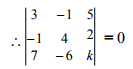Expanding and simplifying, we get k = 8.

Example
21 Find k if the equations x + y + z = 3, x +3y +2z = 6, x +5y +3z = k are inconsistent.

Solution :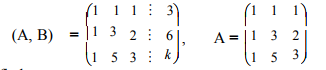We find,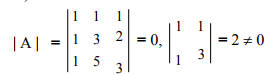Obviously ρ(A) = 2.
For the equations to be inconsistent,ρ(A, B) should not be 2.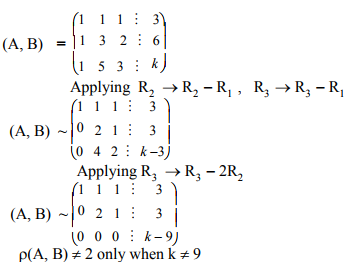∴   The equations are inconsistent when k assumes any real value other than 9.

Example 22
Find the value of k for the equations kx + 3y + z = 0, 3x -4y + 4z = 0, kx - 2y + 3z = 0 to have non trivial solution.

Solution :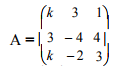For the homogeneous equations to have non trivial solution,
ρ(A) should be less than the number of unknowns viz., 3.
ρ(A) ≠ 3.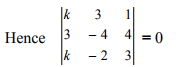Expanding and simplifying, we get k = 11/4

Example 23
Find k if the equations x + 2y +2z = 0, x -3y -3z = 0, 2x +y +kz = 0 have only the trivial solution.

Solution :​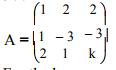For the homogeneous equations to have only the trivial solution, ρ(A) should be equal to the number of unknowns viz., 3.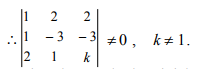The equations have only the trivial solution when k assumes any real value other than 1.

Offer running on EduRev: Apply code STAYHOME200 to get INR 200 off on our premium plan EduRev Infinity!

122 videos|142 docs

,

,

,

,

,

,

,

,

,

,

,

,

,

,

,

,

,

,

,

,

,

,

,

,

;